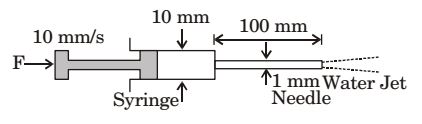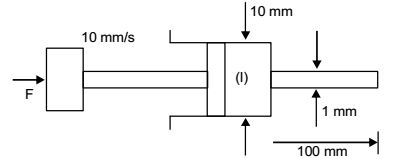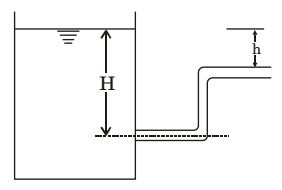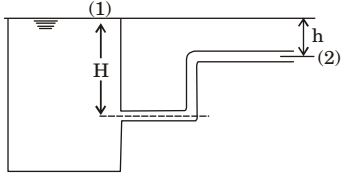## Fluid Mechanics and Hydraulic Machinery Miscellaneous

#### Fluid Mechanics and Hydraulic Machinery

Direction: A syringe with a frictionless plunger contains water and has at its end a 100 mm long needle of 1 mm diameter. The internal diameter of the syringe is 10 mm. Water density is 1000 kg/m3. The plunger is pushed in at 10 mm/s and the water comes out as a jet.1. Assuming ideal flow, the force F in newtons required on the plunger to push out the water is

1. ρwater =1000 kg/m3
Velocity at points 1 = velocity of plunger = 10 mm/ s = 0.014 m/sApplying Bernoulli’s equation at points 1 and 2, we have

 P1 + v1² + z1 = P2 + v2² + z2 ρg 2g ρg 2g

Since z1 = z2 and P2 = 0
 P1 = v2² - v1² ρq 2g 2g

 P1 = ρ (v2² - v1²) ...(i) 2

Applying continuity equation at points (i) and (ii), we have
A1 v1 = A2 v2
 ⇒ v2 =A1v1 A2

 v2 = [ (π / 4) × (0.01)2 ] v1 [ (π / 4) × (0.001)2 ]

= 100 v1 = 100 × 0.01 = 1 m/s
Now from equation (i),
 P1 = 1000 [ (1)² - (0.01)² ] = 499.95 N / m² 2

Force required on plunger = P1 × v1
 = 499.95 × 11 × (0.01)2 = 0.04 N 4

##### Correct Option: B

ρwater =1000 kg/m3
Velocity at points 1 = velocity of plunger = 10 mm/ s = 0.014 m/sApplying Bernoulli’s equation at points 1 and 2, we have

 P1 + v1² + z1 = P2 + v2² + z2 ρg 2g ρg 2g

Since z1 = z2 and P2 = 0
 P1 = v2² - v1² ρq 2g 2g

 P1 = ρ (v2² - v1²) ...(i) 2

Applying continuity equation at points (i) and (ii), we have
A1 v1 = A2 v2
 ⇒ v2 =A1v1 A2

 v2 = [ (π / 4) × (0.01)2 ] v1 [ (π / 4) × (0.001)2 ]

= 100 v1 = 100 × 0.01 = 1 m/s
Now from equation (i),
 P1 = 1000 [ (1)² - (0.01)² ] = 499.95 N / m² 2

Force required on plunger = P1 × v1
 = 499.95 × 11 × (0.01)2 = 0.04 N 4

1. The discharge velocity at the pipe exit in figure is1.By applying Bernoulli’s equation at (1) and (2), we get

 P1 + V1² + Z1 = P2 + V2² + Z2 ρg 2g ρg 2g

P1 = P2 = Patm
V1 = 0
V2 = ?
Z2 = H – h
 ∴ H = V2² + H - h 2g

V2 = √2gh

##### Correct Option: BBy applying Bernoulli’s equation at (1) and (2), we get

 P1 + V1² + Z1 = P2 + V2² + Z2 ρg 2g ρg 2g

P1 = P2 = Patm
V1 = 0
V2 = ?
Z2 = H – h
 ∴ H = V2² + H - h 2g

V2 = √2gh

1. For laminar flow through along pipe, the pressure drop per unit length increases

1.  P1 - P2 = 32μUL D²

##### Correct Option: C

 P1 - P2 = 32μUL D²

1. In fully developed laminar flow in the circular pipe, the head loss due to friction is directly proportional to _____ (mean velocity/square of the mean velocity)

1. f ∝ Mean velocity

##### Correct Option: A

f ∝ Mean velocity

1. Fluid is flowing with an average velocity of V through a pipe of diameter d. Over a length of L,
 the head loss is given by fLV² .The friction factor, f for laminar flow in terms of Reynolds 2gD

number (Re) is _______ (fill in the blanks)

1. For Laminar flow,

 f = 64 Re

##### Correct Option: B

For Laminar flow,

 f = 64 Re